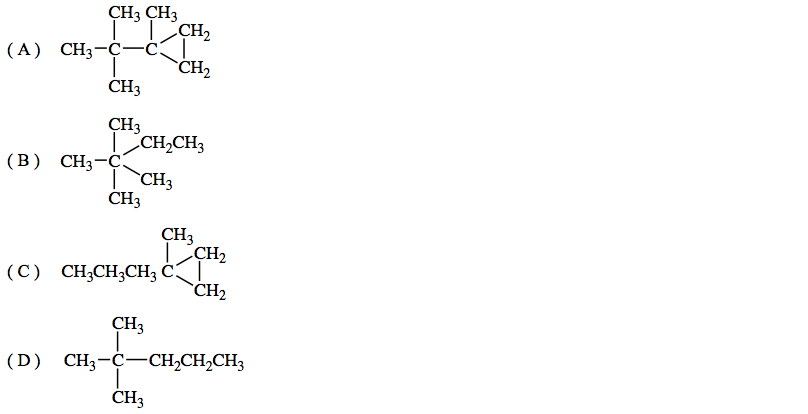Problem: Which formula is equivalent to the condensed formula (CH 3)3C(CH2)2CH3?

FREE Expert Solution
94% (317 ratings)
Problem Details

Which formula is equivalent to the condensed formula (CH 3)3C(CH2)2CH3?What scientific concept do you need to know in order to solve this problem?

Our tutors have indicated that to solve this problem you will need to apply the Condensed Structural Formula concept. You can view video lessons to learn Condensed Structural Formula Or if you need more Condensed Structural Formula practice, you can also practice Condensed Structural Formula practice problems .

What is the difficulty of this problem?

Our tutors rated the difficulty of Which formula is equivalent to the condensed formula (CH 3)3... as low difficulty.

How long does this problem take to solve?

Our expert Organic tutor, Chris took 2 minutes to solve this problem. You can follow their steps in the video explanation above.

What professor is this problem relevant for?

Based on our data, we think this problem is relevant for Professor Daoudi's class at UCF.